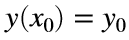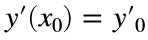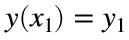# Problem 51387. Solve an ODE: second-order linear equation with constant coefficients

Write a function to solve a second-order linear ordinary differential equation with constant coefficients a, b, and c:with two of the three conditions: (1), (2), and (3). Two of the three elements of the vector bc will be assigned numerical values, and the third will be NaN. The function should return the values of y at the specified values of x.
Equations of this form appear in many applications, such as spring-mass-damper systems, RLC circuits, small-amplitude oscillations of a pendulum, and steady advection, dispersion, and decay of a contaminant in a river or groundwater.

### Solution Stats

20.0% Correct | 80.0% Incorrect
Last Solution submitted on Dec 10, 2023

### Community Treasure Hunt

Find the treasures in MATLAB Central and discover how the community can help you!

Start Hunting!